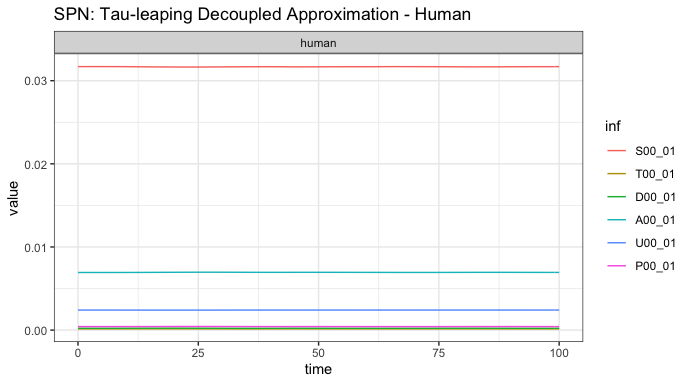# MGDrivE2: One Node Epidemiological Dynamics - Decoupled Imperial Sampling

### Preface

In this vignette, we show how to set up and run MGDrivE2 simulations that utilize the SEI (Susceptible-Exposed-Infectious) mosquito model of epidemiological dynamics coupled to the Imperial human model of epidemiological dynamics. The SEI mosquito model uses an Erlang-distributed incubation (E) period to approximate general non-exponential dwell times in the E state. The Imperial model, originally described in 2010, provides a somewhat complex formulation of malaria transmission dynamics including more states than an SIS approach and population-level immunity. Here we will use an age-structured version, with a plan to expand to biting heterogeneity later on. In this formulation, the stochastic mosquito model is separated from the deterministic, human Imperial model. The two pass data between each other throughout the course of the simulation. See Griffin et al (2010) for a full formulation of the model.

We start by loading the MGDrivE2 package, as well as the MGDrivE package for access to inheritance cubes and ggplot2 for graphical analysis. We will use the basic cube to simulate Mendelian inheritance for this example.

# simulation functions
library(MGDrivE2)
# inheritance patterns
library(MGDrivE)
# plotting
library(ggplot2)

# basic inheritance pattern
cube <- MGDrivE::cubeMendelian()

## Parameterization

Several parameters are necessary to setup the structural properties of the Petri Net, as well as calculate the population distribution at equilibrium, setup initial conditions, and calculate hazards. Again, we specify all entomological parameters as for the mosquito-only simulation (see “MGDrivE2: One Node Lifecycle Dynamics”) as well as additional parameters for the Imperial transmission dynamics. Like the aquatic stages, $$\frac{1}{q_{\mathit{EIP}}}$$ will give the mean dwell time for incubating mosquitoes, and variance by $$\frac{1}{n_{\mathit{EIP}} \cdot q_{\mathit{EIP}}^{2}}$$. In addition to the entomological parameters required by MGDrivE, the set of input parameters to the Imperial model is shown below. The function imperial_model_param_list_create outputs the base set of parameters, and each parameter can be individually modeled. See the source code or appendix text of the provided paper to vary individual parameters. Without any input, the default set of parameters is returned. Then, the function equilibrium_Imperial_decoupled_human calculates the equilibrium values of humans distributed across infection states and age compartments, alongside FOIv, which is used to generate initial conditions in the MGDrivE SPN.

The parameters required by equilibrium_Imperial_decoupled_human are:

Parameter Description
age_vector A vector describing the age compartments from which to build the model
ft The proportion of cases which receive treatment (0 <= ft <= 1)
EIR The desired annual entomological innoculation rate
imperial_params Base parameters for Imperial model, output from imperial_model_param_list_create function

Each mu parameter refers to the mortality rate in that mosquito lifecycle stage. Additionally, we specify a total simulation length of 250 days, with output stored daily. NH, the total number of humans in the simulation, must also be specified.

# generate default set of entomological and epidemiological parameters
theta <- imperial_model_param_list_create()

# age distribution of the population
age_vector <-
c(0,
11 / 12,
1,
4,
5,
14,
15,
59,
60)

ft <- 0.4 # percent of symptomatic cases that are treated

## Initialization of the Petri Net

The Imperial disease transmission model sits “on top” of the existing MGDrivE2 structure, using the default aquatic and male “places”, but expanding adult female “places” to follow an Erlang-distributed pathogen incubation period (called the extrinsic incubation period, EIP). Information on how to choose the proper EIP distribution can be found in the help file for ?makeQ_SEI().

The transitions between states is also expanded, providing transitions for females to progress in infection status and allowing interaction between mosquito and human states through decoupled sampling. All of these additions are handled internally by spn_T_epiSIS_node(). Since only the mosquito portion is stochastic, the SPN will only include the mosquito states. Human states will be handled by the sampling algorithm in the form of a deterministic ODE, the human_Imperial_ODE function.

# Places and transitions
SPN_P <- spn_P_epi_decoupled_node(params = theta, cube = cube)
SPN_T <- spn_T_epi_decoupled_node(spn_P = SPN_P, params = theta, cube = cube)

# Stoichiometry matrix
S <- spn_S(spn_P = SPN_P, spn_T = SPN_T)

## Equilibrium Conditions and Hazard Functions

Now that we have set up the structural properties of the Petri Net, we need to calculate the population distribution at equilibrium and define the initial conditions for the simulation.

The function equilibrium_SEI_decoupled_mosy() calculates the equilibrium distribution of female mosquitoes across SEI stages, based on human populations and force-of-infection, then calculates all other equilibria. We set the logistic form for larval density-dependence in these examples by specify log_dd = TRUE. We also need to augment the cube with genotype specific transmission efficiencies; this allows simulations of gene drive systems that confer pathogen-refractory characteristics to mosquitoes depending on genotype. The specific parameters we want to attach to the cube are b0 and cT, cU, and cD the mosquito to human and human to mosquito transmission efficiencies. We assume that transmission from human to mosquito is not impacted in modified mosquitoes, but mosquito to human transmission is significantly reduced in modified mosquitoes. b0 represents the mosquito-to-human transmission probability in absence of any immunity. cT represents the human-to-mosquito onward infectivity transmission from humans in the T state in the Imperial model (treated but still infectious). Similarly cU represents the onward infectivity from humans in the U state (untreated subpatent infection), and cD represents the onward infectivity from the D state (clinical disease). Each of these must be specified per genotype to characterize reductions in transmission from humans to mosquitoes by different genotypes. This updating of the inheritance cube is handled in equilibrium_Imperial_decoupled.

# Modify parameters with IRS and LLIN coverage
IRS_cov <- 0.2
LLIN_cov <- 0.3

# calculate a target EIR from a given prevalence
prevalence <- 0.7
eir <- convert_prevalence_to_eir(prevalence, age_vector, ft, theta)

# calculate human and mosquito equilibrium
# this function updates theta and the cube and returns initial conditions
eqm <- equilibrium_Imperial_decoupled(age_vector, ft, eir, theta, cube, SPN_P)

# extract updated theta and full set of initial conditions
theta <- eqm$theta cube <- eqm$cube
initialCons <- eqm$initialCons With the equilibrium conditions calculated (see ?equilibrium_SEI_SIS()), and the list of possible transitions provided by spn_T_epiSIS_node(), we can now calculate the rates of those transitions between states. # approximate hazards for continuous approximation approx_hazards <- spn_hazards_decoupled(spn_P = SPN_P, spn_T = SPN_T, cube = cube, params = theta, type = "Imperial", log_dd = TRUE, exact = FALSE, tol = 1e-8, verbose = FALSE) #> --- continue --- ## Simulation of Fully Specified SPN Model ### Deterministic: Decoupled ODE model To first demonstrate that the system is indeed at equilibrium, we show the deterministic solution using decoupled ODE sampling without events. dt <- 1 ode_out <- sim_trajectory_R_decoupled( x0 = initialCons$M0,
h0 = initialCons$H, SPN_P = SPN_P, theta = theta, tmax = 100, inf_labels = SPN_T$inf_labels,
dt = dt,
S = S,
hazards = approx_hazards,
sampler = "ode-decoupled",
events = NULL,
verbose = FALSE,
human_ode = "Imperial",
cube = cube
)

# summarize females/humans by genotype
ode_female <- summarize_females_epi(out = ode_out$state, spn_P = SPN_P) ode_humans <- summarize_humans_epiImperial(out = ode_out$state, index=1)

# plot
ggplot(data = ode_female) +
geom_line(aes(x = time, y = value, color = inf)) +
facet_wrap(~ genotype, scales = "free_y") +
theme_bw() +
ggtitle("SPN: ODE Decoupled Approximation - Mosquito")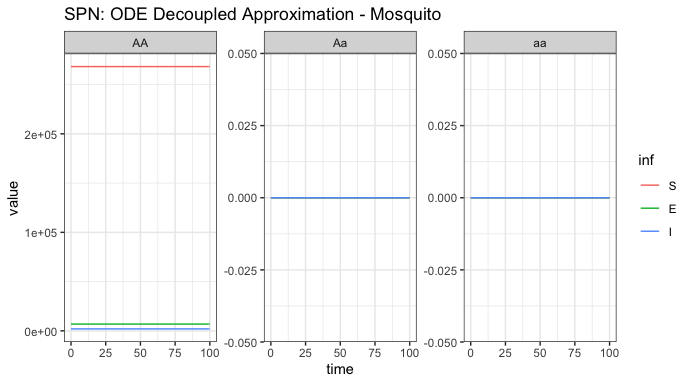ggplot(data = ode_humans) +
geom_line(aes(x = time, y = value, color = inf)) +
facet_wrap(~ genotype, scales = "free_y") +
theme_bw() +
ggtitle("SPN: ODE Decoupled Approximation - Human")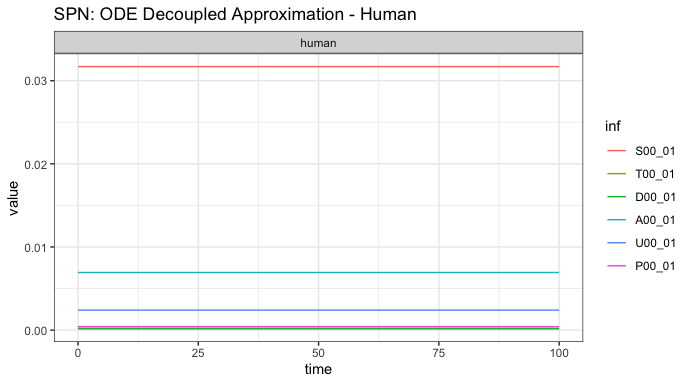### Stochastic: Tau Leaping Solutions {#soln_tau}

As a further example, we run a single stochastic realization of the same simulation, using the tau sampler with $$\Delta t = 1$$, approximating 10 jumps per day. As the adult male mosquitoes do not contribute to infection dynamics, we will only view the adult female mosquito and human dynamics here. We do not show events in this simulation, as genotype-specific dynamics have not yet been implemented.

# delta t - one day
dt_stoch <- 0.1
dt <- 1
# run ode-decoupled simulation
tau_out <- sim_trajectory_R_decoupled(
x0 = initialCons$M0, h0 = initialCons$H,
SPN_P = SPN_P,
theta = theta,
tmax = 100,
inf_labels = SPN_T$inf_labels, dt = dt, dt_stoch = dt_stoch, S = S, hazards = approx_hazards, sampler = "tau-decoupled", events = NULL, verbose = FALSE, human_ode = "Imperial", cube = cube, maxhaz = 1e12 ) # summarize females/humans by genotype tau_female <- summarize_females_epi(out = tau_out$state, spn_P = SPN_P)
tau_humans <- summarize_humans_epiImperial(out = tau_out$state, index=1) # plot ggplot(data = tau_female) + geom_line(aes(x = time, y = value, color = inf)) + facet_wrap(~ genotype, scales = "free_y") + theme_bw() + ggtitle("SPN: Tau-leaping Decoupled Approximation - Mosquito")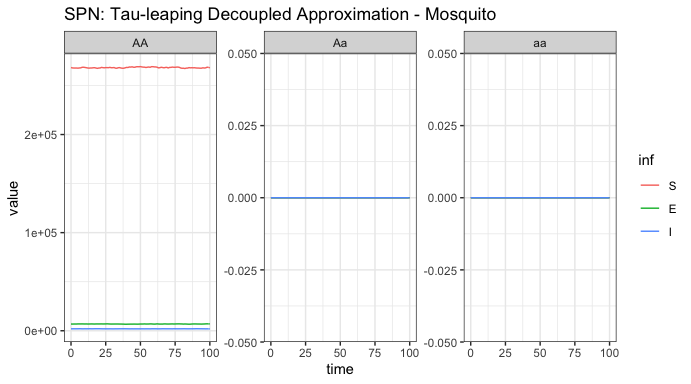ggplot(data = tau_humans) + geom_line(aes(x = time, y = value, color = inf)) + facet_wrap(~ genotype, scales = "free_y") + theme_bw() + ggtitle("SPN: Tau-leaping Decoupled Approximation - Human")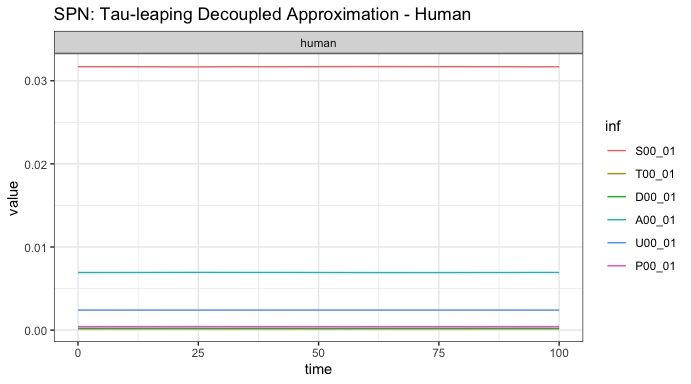In the stochastic simulation, we see that both values drift around equilibrium. Varying delta_t can provide a visual understanding of how the underlying stochastic dynamics converge to the ODE solution as delta_t -> 0. Finally, we show a stochastic simulation with events. Similar to previous simulations, we will release 50 adult females with homozygous recessive alleles 5 times, every 10 days, but starting at day 20. Remember, it is critically important that the event names match a place name in the simulation. The simulation function checks this and will throw an error if the event name does not exist as a place in the simulation. This format is used in MGDrivE2 for consistency with solvers in deSolve. To see the effect on transmission of genotype-specific releases, vary the c and b0 parameters in the inheritance cube (see line 187 of this vignette). r_times <- seq(from = 20, length.out = 5, by = 10) r_size <- 50 events <- data.frame("var" = paste0("F_", cube$releaseType, "_", cube$wildType, "_S"), "time" = r_times, "value" = r_size, "method" = "add", stringsAsFactors = FALSE) tau_out <- sim_trajectory_R_decoupled( x0 = initialCons$M0,
h0 = initialCons$H, SPN_P = SPN_P, theta = theta, tmax = 100, inf_labels = SPN_T$inf_labels,
dt = dt,
dt_stoch = dt_stoch,
S = S,
hazards = approx_hazards,
sampler = "tau-decoupled",
events = events,
verbose = FALSE,
human_ode = "Imperial",
cube = cube,
maxhaz = 1e12
)

# summarize females/humans by genotype
tau_female <- summarize_females_epi(out = tau_out$state, spn_P = SPN_P) tau_humans <- summarize_humans_epiImperial(out = tau_out$state, index=1)

# plot
ggplot(data = tau_female) +
geom_line(aes(x = time, y = value, color = inf)) +
facet_wrap(~ genotype, scales = "free_y") +
theme_bw() +
ggtitle("SPN: Tau-leaping Decoupled Approximation - Mosquito")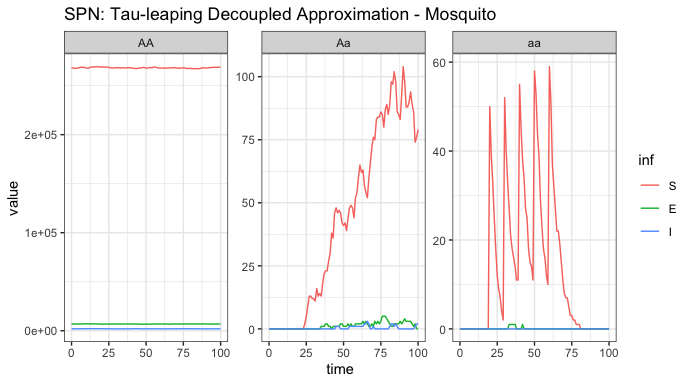ggplot(data = tau_humans) +
geom_line(aes(x = time, y = value, color = inf)) +
facet_wrap(~ genotype, scales = "free_y") +
theme_bw() +
ggtitle("SPN: Tau-leaping Decoupled Approximation - Human")